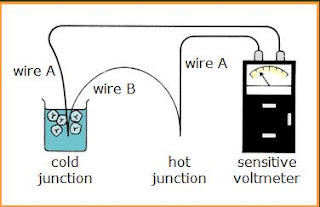## Wednesday, December 31, 2008

### principles of thermometry

What is temperature ?

A measure of the degree of hotness of a body.
is measured using a thermometer

The choice of thermometer depends on
range of temperatures to be measured
accuracy required
physical conditions of the matter concerned

What must all thermometers have?

A physical property that changes with temperature.

Examples of thermometric properties can be……..

expansion of a column of liquid in a capillary tube
the electrical resistance of a platinum wire
the voltage of a thermocouple
the expansion of a bimetallic strip
the pressure of a gas at constant volume

What makes an ideal thermometer ?
safe to use
sensitive to temperature changes
ability to measure a wide range of temperatures

What does calibrating a thermometer mean?

Putting a standard scale on a thermometer,
To do this, two fixed points to be marked are first chosen.
for the purpose of standardisation
always the same under given conditions

How to get the Celsius scale?

The two fixed points for the celsius scale are:

 Ice Point Steam Point Temperature of pure melting ice at standard atmospheric pressure Temperature at which boiling water changes into steam at standard atmospheric pressure Assigned value of 0°C Assigned value of 100°C

Next, the interval between the fixed points is divided into 100 equal divisions for easy reading.
Each reading is 1 degree Celsius (°C)

the Celsius scaleNote height of mercury in the tube at the ice point and the steam point
For any height x of the mercury, the corresponding Celsius temperature  is given bythe Kelvin or absolute scale

The Kelvin scale has its zero at absolute zero
This is the lowest temperature that any substance can reach.

has a SI unit of kelvin (K)
absolute zero corresponds with –273°C on the Celsius scale

T (kelvin) =  (celsius) + 273

thermocouples

consists of two wires of different metals joined together at the end to form two junctions
if the junctions are at different temperatures, a voltage is produced

the larger the temperature difference, the larger the voltage produced
suitable for measuring wide temperature differences, which vary rapidly due to its quick response and the temperature at a point as the wire junctions are very small

the temperature range depend on the two metals used for the wires
can operate over a very wide range of temperatures from –200°C to 1700°C

to produce a larger voltage, several thermocouples are connected in series (thermopiles) to increase the sensitiviy of the instrument#### 1 comment:

1.this is a good way to present the topic. it is easy to understand